Next: Applications Up: Global Optimization Previous: Multi-Resolution Methods and

### The Hough Transform

The Hough transform is well known as a method of determining the parameters of one or more straight lines which pass through a set of points. The lines have parameters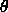and p, and any points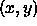on them must satisfy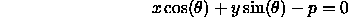However, this equation can also be interpretted as a constraint on the parameters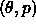to ensure the line passes through the point. Any line passing throughmust have parameterswhich lie on the sinusoid in parameter space determined by the equation above. The Hough approach involves creating an accumulator array in thespace. For every pointwe increment the bins in the array lying on the corresponding sinusoid. We then scan through the array to find the bins with the most entries, which give the parameters of lines through the original points. If suitable noise models are considered when bins are incremented then the distribution of hits in the array about the best entries gives the distribution of parameter values  .

This is a special case of a general Hough approach. In the general case we have a set of N observation vectors,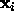, some of which are assumed to satisfy a model equation of the form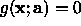, where a specify some model parameters which we would like to determine. Each observation,, imposes a constraint on the parameters a, forcing them to lie on a particular surface in the parameter space. Thus we can determine optimal parameters and their distributions by incrementing the bins of accumulator array which lie under each such surface.

This approach is particularly useful for problems with many observations which are not from the true model, and where there may be multiple instances of the desired model (each leads to a different peak in the accumulator array).Next: Applications Up: Global Optimization Previous: Multi-Resolution Methods and

Bob Fisher
Fri Mar 28 14:12:50 GMT 1997